maths > statistics-basics

Pictograph representation

what you'll learn...

overview

In this page, the pictograph representation of Data is explained with simple examples.

•  A visually pleasing representation of data with pictures is a pictograph.

visually pleasing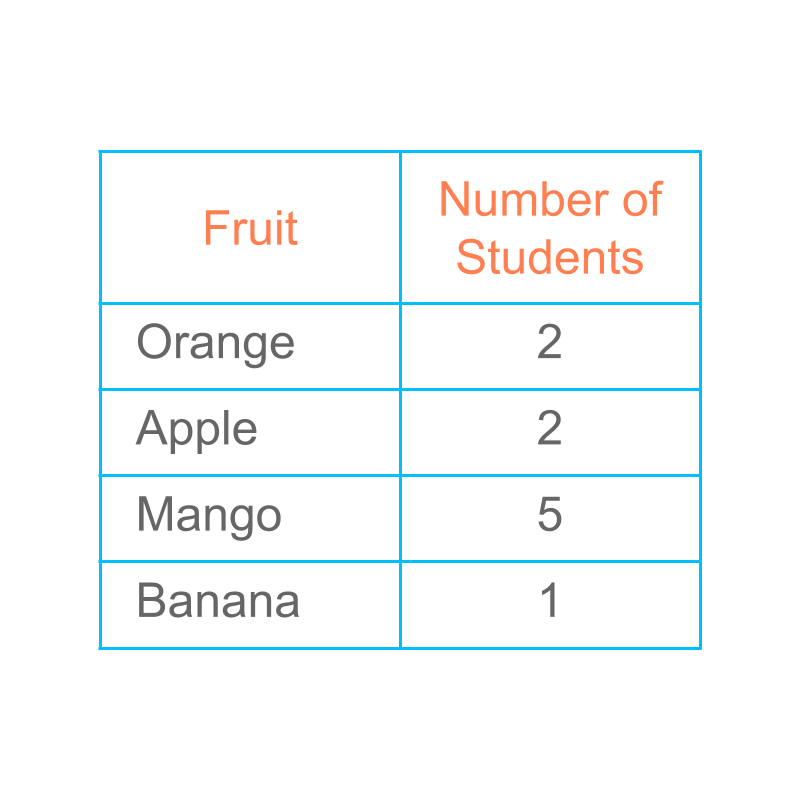We studied that, data can be represented in tally marks or in tabular form.

Such representation is used to understand details like which row is large, which row is empty, etc.

The data can be given using a friendly and artistic form with pictures. It is widely used in newspapers and other similar publications. It is visually pleasing to the readers.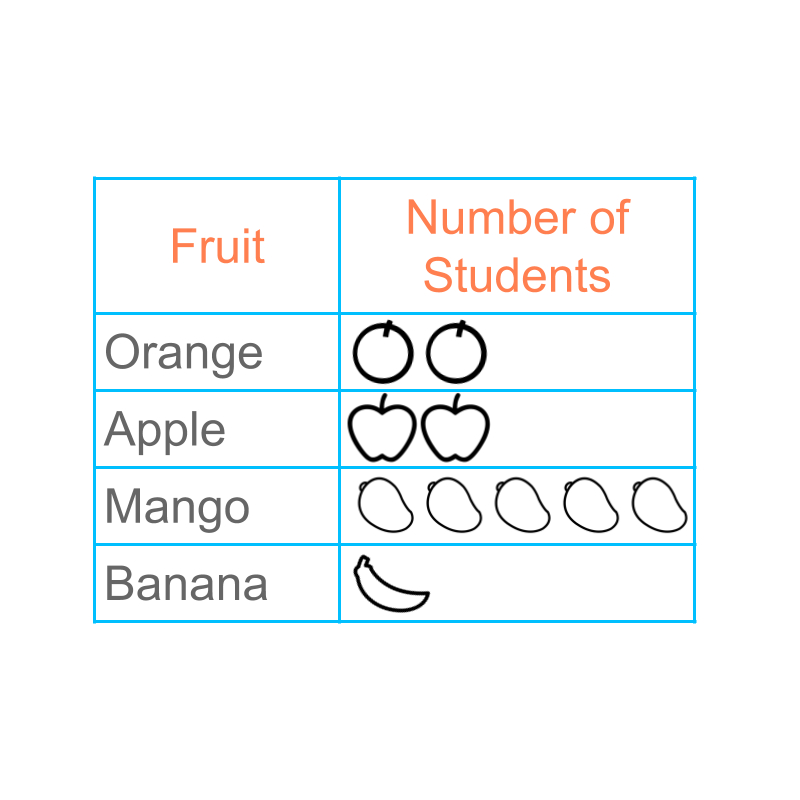Consider the data

orange 2
apple 2
mango 5
banana 1

The figure depicts a "pictograph" of the data.

The word "pictograph" means: data represented with pictures

scale

Earlier, we learned about using tally-marks to represent data. Then, we understood that the tally-marks do not work well for large values in data. This lead to the tabular form of data.

Similarly, the pictograph in the present form will be very hard to represent large values.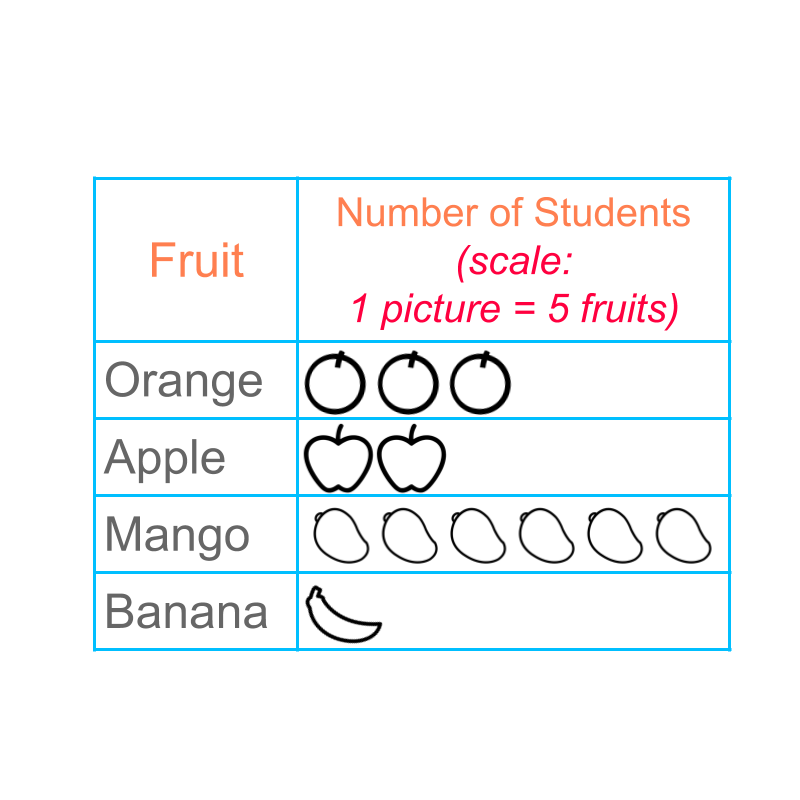Let us consider the data:

Orange : $15$$15$
Apple : $10$$10$
Mango : $30$$30$
Banana : $5$$5$

Instead of representing $1$$1$ fruit with one picture, we can represent $5$$5$ fruits with one picture. A pictograph with scale is given in figure.

Representing $5$$5$ items with one picture is called "scale". The pictograph uses scale of $1:5$$1 : 5$ to represent the data.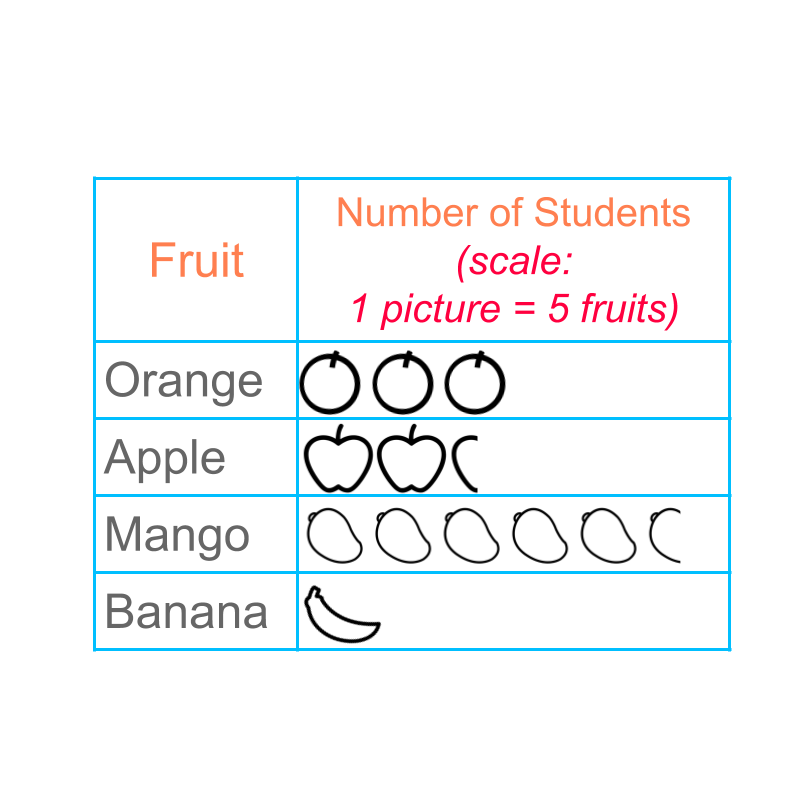A scale of $1:5$$1 : 5$ is used to represent the pictograph.

The number of apples $12$$12$ is represented as $5+5+2$$5 + 5 + 2$, that is two full pictures and one part picture of apple.

Similarly the number of mangoes $28$$28$ is represented.

If a person is provided with the pictograph only, he/she will not be able to find the data accurately. This form of pictograph is not accurate in reproducing the data. Users usually get an idea on which is large, which are comparable, and which is small.

summaryPictograph : A visually pleasing representation of data with pictures is a pictograph.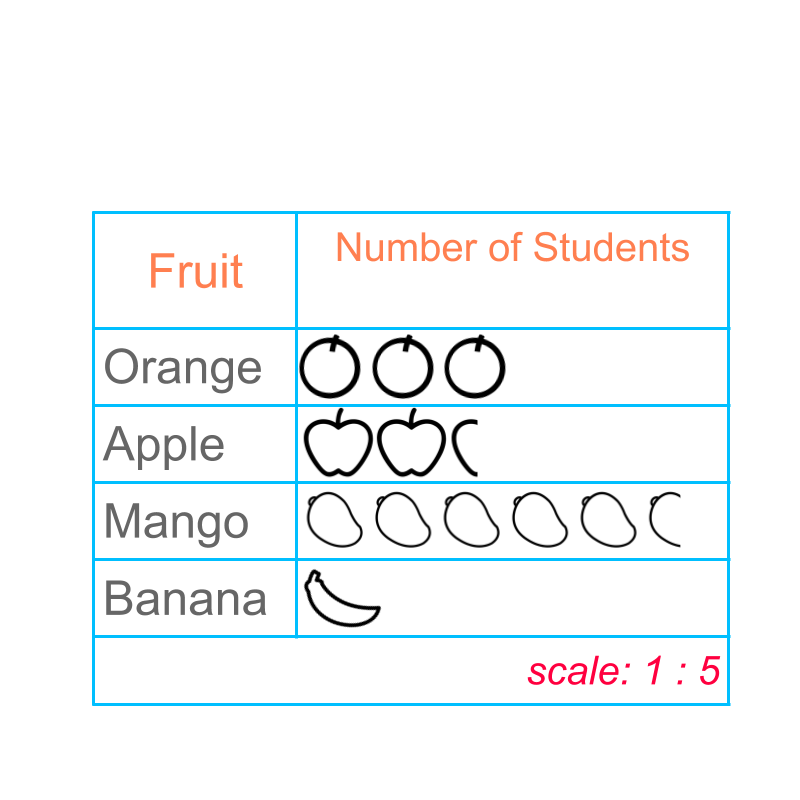Scaling in Pictograph : A pictograph with a specified scale that indicates the number for every picture.

Outline